# Lumberyard Math Libraries: Accuracy Improvements

Authored by David Greer, Senior Engineer on Amazon Lumberyard

Several fundamental mathematical functions in our existing library were calculated using approximations with rather poor accuracy. This post describes how we were able to dramatically improve accuracy without compromising performance.

## Reciprocal Square Root

The first function we turned our attention to was reciprocal square root. This function is very commonly used for normalizing vectors or quaternions. The existing implementation used the `_mm_rsqrt_ps` instruction, which is accurate only to about 11 bits (or roughly 10-3). This led to problems with accuracy, for example the physics team found issues with values passed to the physics backend being rejected as badly normalized.

In the revamped code, we improve the accuracy using the Newton-Raphson method. This is a method for iteratively finding roots of equations which is very powerful because it has quadratic convergence in the neighborhood of the root. Roughly speaking, this means that each iteration doubles the number of correct decimal places in the estimate, so only a single iteration applied to the poor approximation above is enough to obtain an accuracy of around 10-6.

In this case, we are trying to find an improved estimate for y, the reciprocal square root of x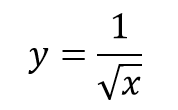Which is equivalent to finding a value of y which satisfies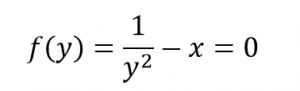The Newton-Raphson method can be applied to get an improved estimate, y’, for the root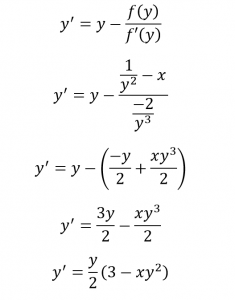So one Newton-Raphson iteration can be applied with inexpensive instructions (4 multiplies and one subtract)

`const __m128 Three = Splat(3.0f);`
`const __m128 Half = Splat(0.5f);`

`const __m128 estimate = SqrtInvEstimate(value);`
`const __m128 estSqValue = Mul(value, Mul(estimate, estimate));`
`return Mul(Mul(Half, estimate), Sub(Three, estSqValue));`

The new version is drastically more accurate, with very little impact on performance. For example, see the table below for a comparison of 1000 calls to `Vector3::GetNormalized` in the two versions.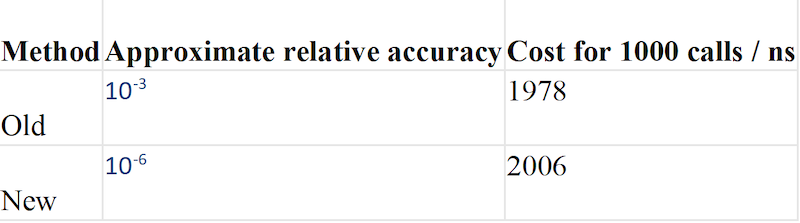## Trigonometric Functions

Another area where we had disappointing accuracy was trigonometric functions such as sine, cosine, tangent and their inverses. These are vital for geometry operations which are ubiquitous in a games engine, but are also fundamental functions which show up in a diverse array of other less obvious applications, such as efficient sampling of normal distributions in random number generation (RNG).

Our existing trigonometric approximations suffered from a couple of major problems. The first was that they used Taylor series type approximations, which are reasonably accurate near the point about which the series is expanded, but tend to become progressively less accurate as we move further away from that point. Secondly, the approximations are computed over unnecessarily large ranges which fail to fully exploit the periodicity of the functions. For example, the plot below shows the relative accuracy (the magnitude of the error divided by the value of the function) for the old sine approximation. The function is approximated over the range [-π, π] (negative values are not shown in the plot but they show symmetrical behavior). The decrease in accuracy moving to the right is evident, with values close to π having a relative error worse than 10-2.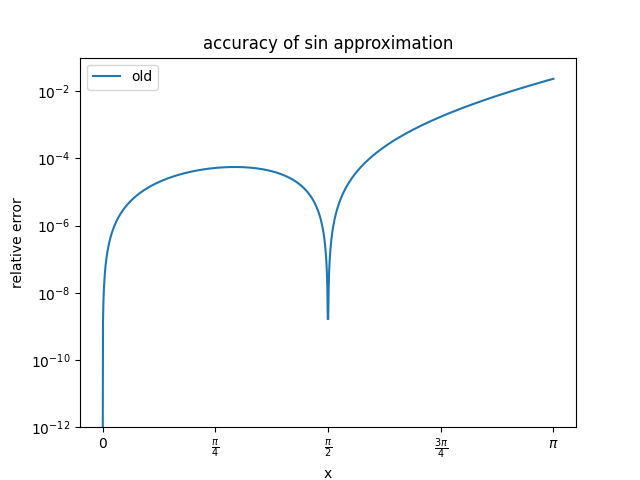We made two key improvements to the approximation based on. The first idea is to use a polynomial approximation which optimizes the worst relative error over the whole interval, rather than concentrating on the neighborhood of a single point. The second is to reduce the size of the interval over which the approximation extends to only cover [-π/4, π/4]. Values outside this range can be computed using trigonometric identities, such as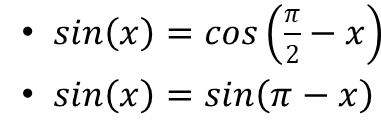Approximating over a smaller interval allows the worst case error to be made much smaller. Putting these ideas together gives an enormous improvement in the worse relative error, as shown below. In fact, the worst relative error of the new method is less than 10-7, more than 5 orders of magnitude more accurate.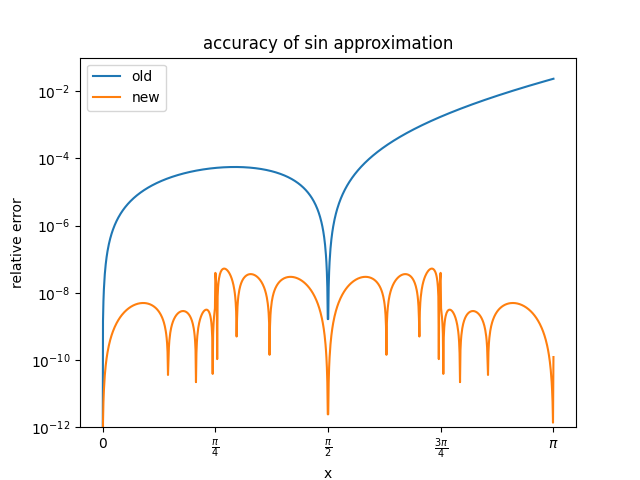However the new method still uses the same order of polynomial for its approximation, so is no more expensive to compute. Actually, by using a more optimized approach for performing the range reduction step which avoids an expensive Calculate Floating Point Remainder instruction, the new code is significantly faster. On top of that, even though it used SIMD instructions, the old method could only compute the sine and cosine for a single value at a time. The new code can operate on up to 4 values at once, giving a further 4x improvement for use cases which are parallelizable.

The other trigonometric functions apply similar principles of range reduction and optimizing the error over the entire interval to achieve similar improvements in accuracy, with performance improving likewise.

Sources

 https://software.intel.com/sites/landingpage/IntrinsicsGuide/
 Riley, K., Hobson, M., & Bence, S. (2002). Mathematical Methods for Physics and Engineering: A Comprehensive Guide (2nd ed.). Cambridge: Cambridge University Press. doi:10.1017/CBO9781139164979
 Robin Green (2008). More Approximations to Trigonometric Functions. In DeLoura, M. Best of Games Programming Gems. Charles River Media, Inc., USA.